# Tackling Troublesome Problems

Problem

You're dealing with a particularly troublesome optimization problem where you can't seem to converge on a global optimum. For example, Solver converges to several different solutions given different initial values. You suspect Solver is converging to local optima rather than a true global optimum solution.

Solution

Getting trapped by local maxima or minima is an unfortunate part of optimization. Some problems are just riddled with local extrema over their objective function landscape and, depending on your assumed initial guess, you may end up in one of these locally optimum solutions rather than finding a globally optimum solution. One way around this is to select different initial guesses just as I discussed in Chapter 9 when finding multiple roots of a nonlinear equation. Also, if possible, you should plot the objective function to get a feel for how it behaves. This is not always possible for multidimensional problems, though.

As an alternative, you can use a Monte Carlo-inspired approach by repeatedly using Solver for many initial guesses. The following discussion shows you how.

Discussion

Take a look at the function plotted in Figure 13-9.

Figure 13-9. Troublesome function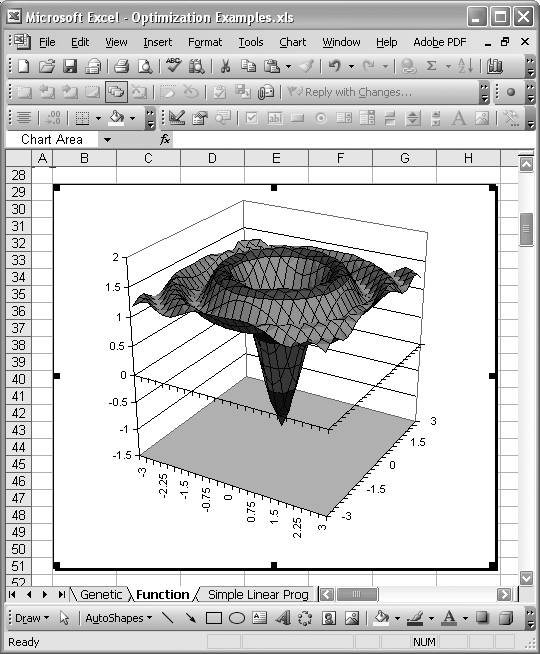This function wreaks havoc on Solver. The equation for this function is: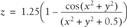Let's look at this function from a different angle. Check out Figure 13-10.

Figure 13-10. Cross-section of troublesome function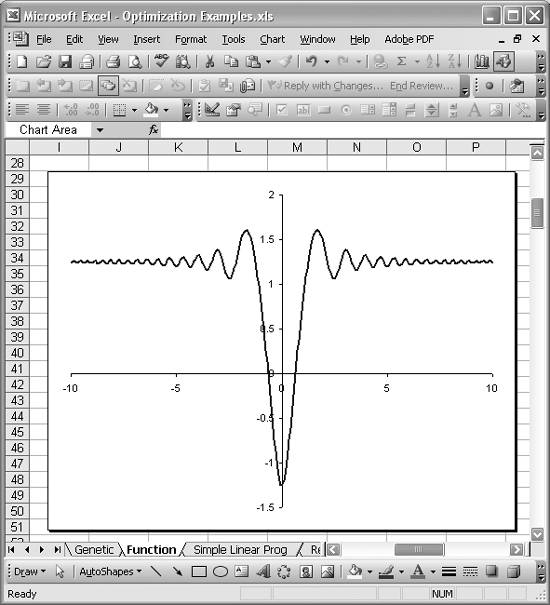The shape of this function provides for an infinite number of local extrema that can trap Solver. It's a veritable mine field of local optima! For example, I ran Solver to minimize this function by varying x and y and with an initial (x,y) = (5,8). Solver found the "minimum" z = 1.236 corresponding to (x, y) = (4.971, 7.953). Obviously that's wrong, since you can see clearly from the two charts of this function that the minimum z is -1.25 at (x, y) = (0, 0). Trying another initial guess for (x, y) didn't help matters. An initial guess of (x, y) = (10, 3) yielded a minimum z of 1.238; another initial guess of (x, y) = (2, 1) yielded a minimum z of 1.064; yet another initial guess of (x, y) = (1.5, 1.5) yielded a minimum z of 1.064. All of these are wrong!

Since you can plot this function, you can see where the global minimum lies and choose much better initial values for x and yvalues close to (0, 0). But what if this was a higher-dimensional problem that you could not plot? If you were trying to minimize such a function, you'd very likely get stuck in a local minimum unless you were lucky enough to pick just the right initial guess. Therefore, it would be prudent for you to try many different guesses in an effort to comprehensively cover the possible solution domain, homing in on the global optimum. You can indeed perform such an exercise using Solver manually, but that would get dull rather quickly.

A better approach, inspired by Monte Carlo methods, involves programmatically selecting many random initial guesses and running Solver for each guess. Such sampling of the solution domain would allow you to quickly evaluate many possible solutions. This approach is easy to program in Excel, since you can call Solver directly from VBA code (see Recipe 9.3). I'll show you how to do it.

Figure 13-11 shows a simple spreadsheet I set up for this problem.

Figure 13-11. Monte Carlo-inspired solution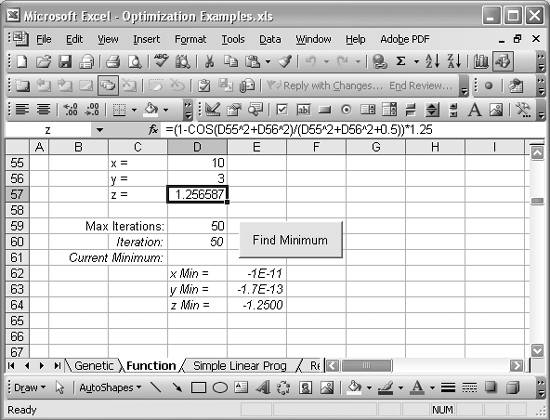Cell D57 contains the formula for our objective function: =(1 - COS(D55^2+D56^2) / (D55^2+D56^2+0.5)) * 1.25. The formula is also shown in the formula bar in Figure 13-11, since cell D57 is selected in that figure. Cells D55 and D56 contain the initial guesses for x and y.

Cell D59 contains a value for the maximum number of iterations that will be used by the VBA subroutine I'll show you shortly. I'll explain this more in a moment. The other cells, formatted with italic text, are status messages generated by the VBA subroutine I'll show you. These status messages will allow you to see how the iterative solution is progressing. The button you see in Figure 13-11 will launch the iterative subroutine when you click it. See Recipe 9.3 to learn how to add this sort of button.

The iterative solution I want to show you involves generating many random initial guesses for x and y within the solution domain for this problem. These guesses, or random samples, will then be used as initial guesses for running Solver. Actually, the algorithm I'll show you takes a random sample, runs Solver, and stores the result. Then it picks another random sample, runs Solver again, and compares the new result with the previous one. If the new result, the minimum z, is lower than the previous one, then we know the previous result was only a local minimum, so we discard it and replace it with the new one. This process repeats over and over until the maximum number of iterations that you specified in cell D59 is reached.

Example 13-1 shows the VBA subroutine I wrote that implements this algorithm.

Example 13-1. Algorithm to iteratively minimize a function

 ```Private Sub FindMinimumButton_Click( ) ' Declare local variables: Dim xMin As Double Dim yMin As Double Dim zMin As Double Dim n As Integer Dim Z As Double Dim MaxIterations As Integer With Worksheets("Function") ' Initialize variables: n = 0 xMin = .Range("x") yMin = .Range("y") zMin = .Range("z") MaxIterations = .Range("MaxIterations") ' Randomize the random number generator: Randomize ' Generate random values for x and y: .Range("x") = (5 - (-5) + 1) * Rnd + (-5) .Range("y") = (5 - (-5) + 1) * Rnd + (-5) ' Start solution iterations: Do While (n <= MaxIterations) .Range("Iteration") = n ' Run Solver: SolverOK SetCell:=.Range("z"), MaxMinVal:=2, ByChange:=.Range("x", "y") SolverSolve UserFinish:=True SolverFinish KeepFinal:=1 Z = .Range("z") ' Check result: If Z < zMin Then xMin = .Range("x") yMin = .Range("y") zMin = Z .Range("x_Min") = xMin .Range("y_Min") = yMin .Range("z_Min") = zMin End If ' Generate random values for x and y: .Range("x") = (5 - (-5) + 1) * Rnd + (-5) .Range("y") = (5 - (-5) + 1) * Rnd + (-5) n = n + 1 Loop .Range("x") = xMin .Range("y") = yMin End With End Sub ```

I've commented the code so you can generally see what's going on. The first several lines in the subroutine declare local variables in the usual manner. xMin, yMin, and zMin store the current minimum x, y, and z as the routine iterates over many trial values. n is just a counter variable used to track the current iteration. z stores the z value found by Solver in each iteration; it may or may not be the global minimum z. Finally, MaxIterations stores the maximum number of iterations you set in the spreadsheet (cell D59).

Upon entering the With statement (see Recipe 9.3) the routine initializes several variables. Basically, the counter n is set to 0, while the other variables mentioned earlier are set to values extracted from the worksheet named Function.

Randomize is then called to initialize VBA's random number generator. Next, two random numbers between the bounds -5 and 5 are generated and stored in cells D55 and D56, named x and y, respectively. These serve as random initial guesses before we call Solver.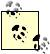See Recipe 5.8 to learn how to use Excel's and VBA's random number functions. Also, see Recipe 1.14 to learn more about cell names.

Next, a Do While loop is entered to iterate MaxIterations times, generating random initial guesses and calling Solver each time. Let's look inside the Do While statement.

The line .Range("Iteration") = n sets the value of cell D60, named Iteration, to n. This provides feedback to you on the status of the subroutine as it runs. The next several lines invoke Solver as described in Recipe 9.3. In this case, the target cell is set to the cell named z, and the cells to change are the ones named x and y. MaxMinVal is set to 2, indicating that we want to minimize the target cell. When Solver is finished, the cell named z in the worksheet named Function, should contain a minimum of the subject function. However, this may or may not be the global minimum. So, we have to check it against the currently stored minimum, zMin.

First, the value from the cell named z is extracted and stored in variable z. The following If statement checks whether or not z is less than the stored zMin. If it is, then we found a better estimate of the minimum of the subject function, which should be saved in the current minimum variables. The three lines xMin = .Range("x"), yMin = .Range("y"), and zMin = Z take care of this task. The next three lines echo the current minimum variables back to the spreadsheet so you can see their status as the routine iterates.

Upon exiting this If statement, the routine generates two new random guesses for x and y and increments the iteration counter by 1. The Do While loop then repeats until MaxIterations is reached.

After the maximum number of iterations has been reached, the minimum values found are echoed back to the spreadsheet.

When the routine is finished, cells E62 through E64 will contain the minimum z-value and corresponding x- and y-values for the subject function. After running this routine many times, I have found that it finds the global minimum in all but a very few cases; in those cases, a randomly selected initial guess that would have converged toward the global minimum was never selected. You can reduce the likelihood of that occurring by cranking up the value for MaxIterations.

The approach shown in this recipe goes a long way to mitigate getting stuck at local minima or maxima. It's not the only approach, though. Take a look at Recipe 13.7 for a completely different approach.Excel Scientific and Engineering Cookbook (Cookbooks (OReilly))
ISBN: 0596008791
EAN: 2147483647
Year: N/A
Pages: 206
Authors: David M Bourg